Ask your WordPress questions! Pay money and get answers fast!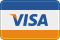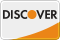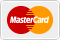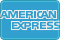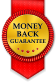# How to explode csv string safely into a usable php array WordPress

• SOLVED

My code below is wrong. I need help fixing it.

```// rider nationality function motocom_rider_nationality( \$field ) { // reset choices \$field['choices'] = array(); // get the textarea value from options page without any formatting \$choices = get_field('nationality_codes', 'option', false); // explode the value so that each line is a new array piece \$choices = explode(",", \$choices); // remove any unwanted white space \$choices = array_map('trim', \$choices); \$field['choices'] = array( null => 'Select nationality...' ); // loop through array and add to field 'choices' if( is_array(\$choices) ) { foreach( \$choices as \$choice ) { \$label = \$choice['Country']; \$value = \$choice['A4']; \$field['choices'][ \$value ] = \$label; } } // Important: return the field return \$field; } add_filter('acf/load_field/name=rider_nationality', 'motocom_rider_nationality');```

I have a field that contains a string. The string is in the format of a comma delimetered csv file.

See the first few lines of csv including header row below...

```Country,A2,A3,Number Aaland Islands,AX,ALA,248 Afghanistan,AF,AFG,4 Albania,AL,ALB,8 Algeria,DZ,DZA,12 Samoa,AS,ASM,16 Andorra,AD,AND,20```

What I am trying to achieve is for the field choice value and label to use data from the csv.

```\$label = \$choice['Country']; \$value = \$choice['A4'];```

See for the \$label I would like the Country column to be used. And the \$value to use A4 column.

Can any one help fix my function please?

Thanks

-------

Finally got my original code working...

``` // csv to array function csv_to_array(\$filename='', \$delimiter=',') { if(!file_exists(\$filename) || !is_readable(\$filename)) return FALSE; \$header = NULL; \$data = array(); if ((\$handle = fopen(\$filename, 'r')) !== FALSE) { while ((\$row = fgetcsv(\$handle, 1000, \$delimiter)) !== FALSE) { if(!\$header) \$header = \$row; else \$data[] = array_combine(\$header, \$row); } fclose(\$handle); } return \$data; } // rider nationality function motocom_rider_nationality( \$field ) { // reset choices \$field['choices'] = array(); // get the textarea value from options page without any formatting \$choices = csv_to_array( get_template_directory() . '/csv/nationality-codes.csv' ); \$field['choices'] = array( null => 'Select nationality...' ); // loop through array and add to field 'choices' if( is_array(\$choices) ) { foreach( \$choices as \$choice ) { \$label = \$choice['Country']; \$value = \$choice['A3']; \$field['choices'][ \$value ] = \$label . ' [' . \$value . ']'; } } // Important: return the field return \$field; } add_filter('acf/load_field/name=rider_nationality', 'motocom_rider_nationality'); ```

2014-02-24

Hello,

"\$choices" have values or null? Let me know

`\$choices = get_field('nationality_codes', 'option', false);`

will contain this...

```Country,A2,A3,Number Aaland Islands,AX,ALA,248 Afghanistan,AF,AFG,4 Albania,AL,ALB,8 Algeria,DZ,DZA,12 Samoa,AS,ASM,16 Andorra,AD,AND,20```

But could be empty then will only show

`null => 'Select nationality...'`

No the array is not working correctly, see result here.

http://imgur.com/MBG89UO

Change it like this
``` // get the textarea value from options page without any formatting \$choices = unserialize(get_field('nationality_codes', 'option', false));```

Now the results are empty. Not working now.

Okay remove that code. Let me know what you when trying following code

``` // get the textarea value from options page without any formatting \$choices = get_field('nationality_codes', 'option', false); echo \$choices ; // explode the value so that each line is a new array piece \$choices = explode(",", \$choices); print_r(\$choices); ```

Don't worry I've fixed it.

Thanks your help. Will spilt the pot.

2014-02-24

try this
``` foreach( \$choices as \$choice ) { \$label = \$choice['Country']; \$value = \$choice['Number']; \$field['choices'][ \$value ] = \$label; }```

change \$value = \$choice['A4']; to \$value = \$choice['Number'];

No the array is not working correctly, see result here.

http://imgur.com/MBG89UO

Don't worry I've fixed it.

Thanks your help. Will spilt the pot.

2014-02-24

You can also change it with:

``` \$label = \$choice; \$value = \$choice; ```

Nope - the array is not splitting properly...

http://imgur.com/MBG89UO